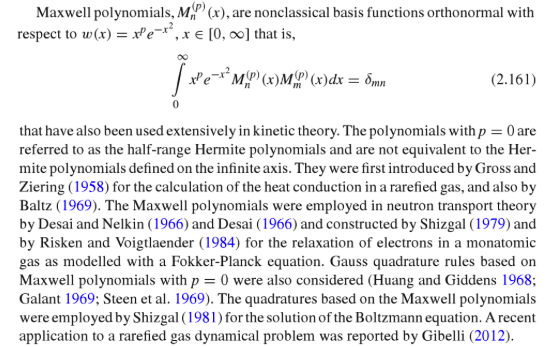# What are the orthogonal polynomials w.r.t. Maxwell distribution

Is there a way to get a clean presentation of the orthogonal polynomials w.r.t. the Maxwell distribution https://en.wikipedia.org/wiki/Maxwell%E2%80%93Boltzmann_distribution ? If you separate the problems to an even and odd problems, the even part of the orthogonal basis behaves nice enough: one simply takes the quotient of the odd Hermite polynomials by x (since the Maxwell distribution is given by $Cx^2\exp(-x^2/2)$, and Hermite polynomials are orthogonal w.r.t. $\exp(-x^2/2)$) ; as for the even part, I described a semi-solution in the comment below. However, I have no ide how the two parts interact.

The analog question for Laguerre polynomials (i.e. when one moves from the measure $\exp(-x)$ to the measure $C_{\alpha}x^{\alpha}\exp(-x)$ yields a very satisfying result in the form of generalized Laguerre polynomials. Due to the relation between Laguerre polynomials and Hermite polynomials, I'd expect something similar here.

• For what it's worth, setting $F_{2n+1}=(H_{2n+2} + (2n+1)H_{2n})/x$ for the odd degree polynomials, (which - as mentioned in the question - are the only problem), the inner products form a symmetric tri-diagonal matrix. – David Lehavi Jun 12 '16 at 15:17
• According to this reference link.springer.com/chapter/10.1007/978-94-017-9454-1_2 page 30, these are Sonine polynomials with $\alpha=1/2$. But I am afraid that these are only polynomials in $x^2$, that is not what you are asking about. – Fedor Petrov Jun 14 '16 at 9:46
• @FP, thanks, but indeed I want the full orthogonal basis - odd powers included - and I can't believe I'm the first one asking this question, it's very natural if you are analyzing random points in 3D. – David Lehavi Jun 14 '16 at 9:53

These are the socalled Maxwell polynomials $$M_n^{(p)}(x)$$, see page 75 and following of Spectral Methods in Chemistry and Physics. The coefficients for $$p=2$$, which is the case you need, are given in Table 2.14 of that reference.